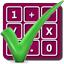#### Arithmetic of Computers

#### from Tenscope Limited

By using this site, you are accepting "session" cookies, as set out in the site's Privacy Policy
Cookies are also used to remember which page of the book you last viewed, so that when you revisit the site you automatically return to the last page you visited.

### Lesson 5

#### Page 154

We have seen that numbers can be represented by adding together multiples of powers of a particular base number. For example, we can represent the number 54 as a sum of muliples of powers of 4:
3(42) + 1(41) + 2(40)  = 3(16) + 1(4) + 2(1)  = 48 + 4 + 2 = 54.
We also know the names for the parts of the expression that are in the parentheses, as shown below:
exponents 3 (42)  + 1 (41)  + 2 (40) base
In each of the terms written within parentheses, the number that is to be used as a factor (4 in this case) is called the base, and the number that tells how many times the base is to be used as a factor is called the exponent. This is all familiar.
The numbers outside the parentheses in the expression above have a special name, too. These multipliers are called coefficients. In our example here the coefficients are 3, 1 and 2.
3 (42)  + 1 (41)  + 2 (40) coefficients
In the expression 4(103) + 9(102) + 2(101) + 1(100) what is the coefficient of 101?